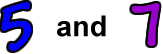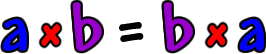How about trying the same thing with some other numbers:Do we get the same thing when we multiply them...  even if we switch the order?Yep.  Sure looks like it!

I want you to try it now...  Go ahead...  Pick any two numbers you want...  Multiply them together...  Then, switch the order and multiply them again!  I'll wait...

Did you get the same thing?  You always will.  When something happens like this in math, we make it a special rule called a "property."

Here's the property in all letters to get you ready for Algebra!

 The Commutative Property of Multiplication:Where a and b are any real numbers.

This rule just says that, when you are doing multiplication, it doesn't matter which order the numbers are in.  You can multiply a and b OR you can multiply b and a ... and you'll  get the same answer.

NOTE:  This does not work with division!